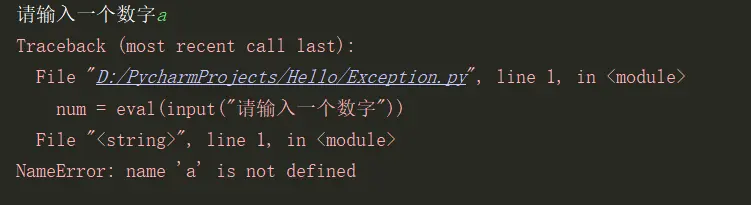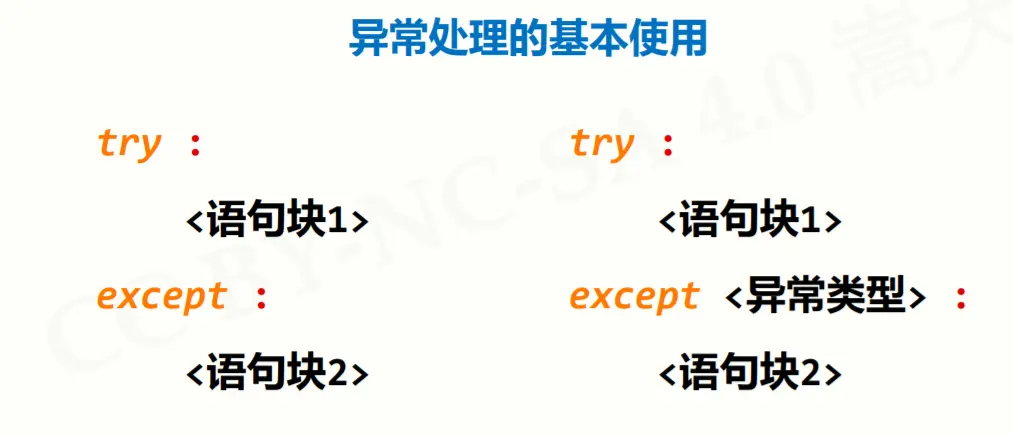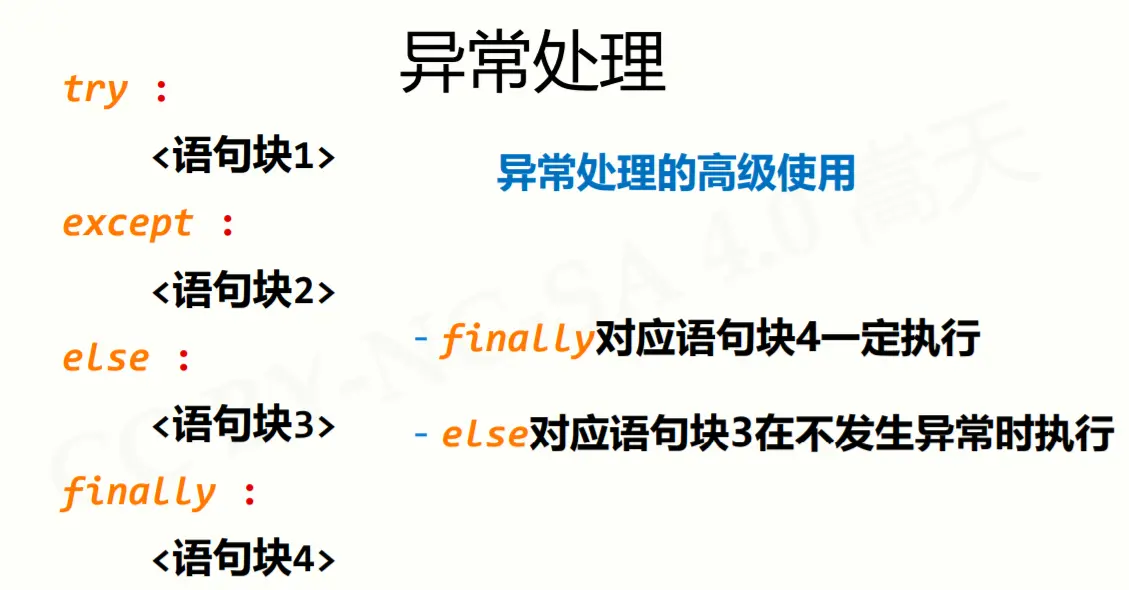# Python 中的异常及其处理

• 时间:

1、为什么要有异常处理呢？

``````num = eval(input("请输入一个数字"))
print(num ** 2)
````````````num = eval(input("请输入一个数字"))
if(num 是一个数字):
print(num ** 2)
else:
print("您输入的不是数字")
``````

``````try:
num = eval(input("请输入一个数字"))
print(num ** 2)
except:
print("您输入的不是数字")
``````2、异常处理的基本使用``````try:
num = eval(input("请输入一个数字"))
print(num ** 2)
except:
print("您输入的不是数字")
``````

``````try:
num = eval(input("请输入一个数字"))
print(num ** 2)
except NameError:
print("您输入的不是数字")
``````

3、高级的异常处理else语句是当程序没有发生异常，我们作为奖励代码，额外执行，finally为一定执行的代码（无论是否发生异常）